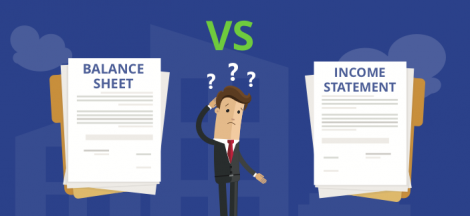In a statistical experiment, probability determines the outcome unlike the experiment from physical sciences where you will always get the same result as long as the experiment is repeated in exactly the same way. Experiments in statistics are called random experiments.

An experiment is any process that generates well defined outcomes. These experimental outcomes are known as Sample space and every individual outcome is a sample point. For example, a tossed coin is an experiment, the head or tail outcome is the sample space while the individual outcome which is a head or a tail is the sample point.

Probability is a numerical measure of the likelihood that an event will occur. An event is a collection of sample points and the probability of any event is equal to the sum of the probability of the sample points in that event.Probability values are placed on a scale between 0 to 1. A probability value that moves closer to 0 shows that the event is less likely to occur while that that moves closely to 1 is almost certain to occur. A probability value of 0.5 shows that the occurrence of the event is just as likely to occur as it is unlikely to happen.

There are three methods for  assigning a probability and they include:

1. Classical method: assigning probability using this method is based on the assumption of having equally likely outcomes. For example, if a die is rolled once, the probability of having any of the numbers as a likely outcome is equal.
2. Relative Frequency Method: This method assigns probability based on experimental and historical data
3. Subjective Method: This has to do with assigning probabilities based on judgement or from experience.

There are some basic probability relationships that can be used to compute the probability of an event when we do not have knowledge of all the sample points probability. These relationships are:

1. Complement of an event: The complement of an event A, consists of all sample point that see not in A. It is denoted by AC
2. Addition law  is used to compute the probability of of event A or B, or both A and B occurring
• Union of Events: The union of event A and B is the event containing all sample points that are in A or B or both.It is denoted by A u B
• Intersection of Events: The intersection of events A and B is the set of all sample points that are in both A and B. This is denoted by A u B

Addition law provides a way to compute the probability of event A or B or both. The law is written as P(A U B) = P(A) + P(B) – P(A n B)

1. Conditional probability: is the probability of an event occurring given that another event has occurred. The conditional probability of A occurring, given that B has occurred is denoted as: P(A | B). It is represented as P( A| B) = P(A n B) / P(B)
2. Multiplication law : To make the intersection the subject formula from conditional probability, we will have P(A n B) = P(B)* P(A | B)## Understanding the Balance Sheet and the Income Statement: A Guide for Business Managers #MMBA5

in